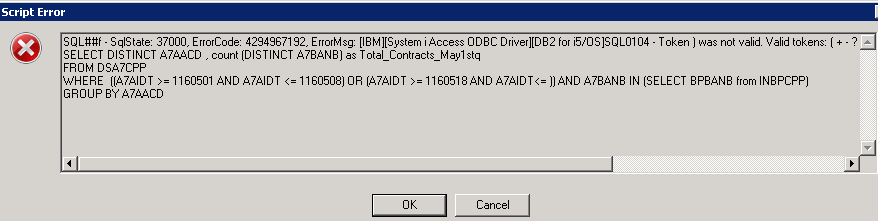# New to QlikView

Discussion board where members can get started with QlikView.

Announcements
Attend QlikWorld 2020 and hear keynote speaker, Malcolm Gladwell. \$300 savings extended to February 9th Learn More
Highlighted
Contributor III

## Excluding date range with in date range

Hi,

Currently we were capturing the data from April 1st to till date.We have hard coded the start date means April 1st as an variable as

VstartDate = 11060401 and Vcurrentdate as 11060609 then we use these variables in the logic to get the data..

Now we got an change request to exculde the date range like they want to exculde the data in between 26th april to 30th April..

AS: Sum(sales) from 11060401 to 11060609 (Excuding sum(sales) from 11060426 to 11060430).

date field : Sales_Date

Thanks...

Tags (1)
1 Solution

Accepted SolutionsMVP

## Re: Excluding date range with in date range

May be like this:

SELECT A.A7AACD ,B.BBR2TT as BBR2TT_MTD_Mule , COUNT(DISTINCT B.BBBANB) as count_based_on_mule_status_MTD

FROM DSA7CPP A ,  INBBCPP

B WHERE A.A7BANB = B.BBBANB AND ((A.A7AIDT >= 11060401 AND A.A7AIDT <= 11060426) OR (A.A7AIDT >= 11060430 AND A.A7AIDT<= \$(vyesterday)))

GROUP BY A.A7AACD,B.BBR2TT ;

8 RepliesMVP

## Re: Excluding date range with in date range

May be like this:

Sum({<VstartDate = {'>=11060401<=11060426', '>=11060609<=11060430'}>}sales)

Sum({<VstartDate = {'>=11060401<=11060426', '>=11060430<=11060609'}>}sales)MVP

## Re: Excluding date range with in date range

or this:

Sum({<VstartDate = {'>=11060401<=11060609'}-{'>=11060426<=11060430'}>}sales)

Contributor III

## Re: Excluding date range with in date range

Hi,

We have filter the data at script level...

below is the script..

SELECT A.A7AACD ,B.BBR2TT as BBR2TT_MTD_Mule , COUNT(DISTINCT B.BBBANB) as count_based_on_mule_status_MTD

FROM DSA7CPP A ,  INBBCPP

B WHERE A.A7BANB = B.BBBANB AND A.A7AIDT >= \$(11060401) AND A.A7AIDT <= \$(vyesterday)

GROUP BY A.A7AACD,B.BBR2TT ;

Where vYesterday = today()-1

So in between from 11060401 to vyesterday ineed to exculde the data from 11060426 to 11060430.

Thanks..

Contributor III

## Re: Excluding date range with in date range

Hi,

Any suggestions...MVP

## Re: Excluding date range with in date range

May be like this:

SELECT A.A7AACD ,B.BBR2TT as BBR2TT_MTD_Mule , COUNT(DISTINCT B.BBBANB) as count_based_on_mule_status_MTD

FROM DSA7CPP A ,  INBBCPP

B WHERE A.A7BANB = B.BBBANB AND ((A.A7AIDT >= 11060401 AND A.A7AIDT <= 11060426) OR (A.A7AIDT >= 11060430 AND A.A7AIDT<= \$(vyesterday)))

GROUP BY A.A7AACD,B.BBR2TT ;

Contributor III

## Re: Excluding date range with in date range

Hi,

I got the below error after the script run..Thanks..MVP

## Re: Excluding date range with in date range

Seems like an issue with your variable here. Was it working before we added the new code (the variable I mean)?

Contributor III

## Re: Excluding date range with in date range

Thanks..

Issue with the variable.

Issue got resolved thanks..